# 如何用Neo4j和Scikit-Learn做机器学习任务？| 附超详细分步教程

Neo4J 主要基于Cypher语言，基于Graph Algorithm 实现图分析算法。获取安装Neo4j Desktop也非常容易，只需一键。

Neo4j Desktop 地址：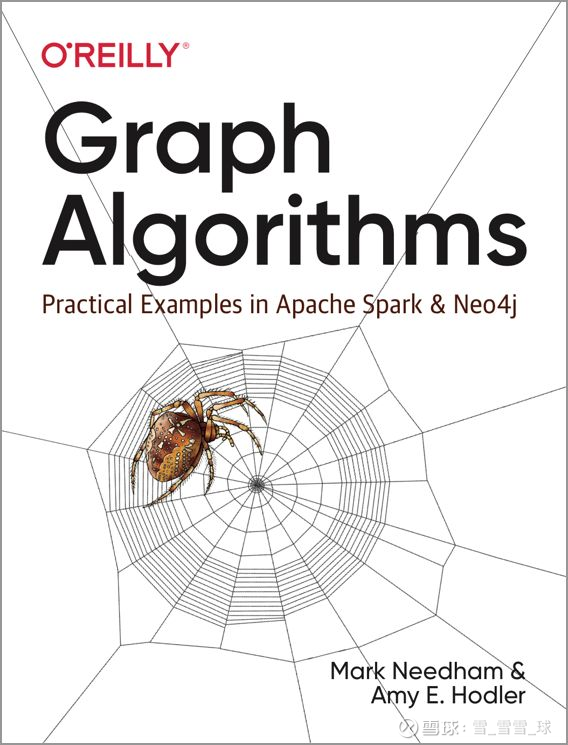（一）什么是链路预测？

（二）链路预测算法

Kleinberg 和 Liben-Nowell 介绍了一系列可以用于链路预测的算法，如下图所示：Kleinberg 和 Liben-Nowell 在论文中所介绍的算法

（三）算法评估标准

1、共同邻居数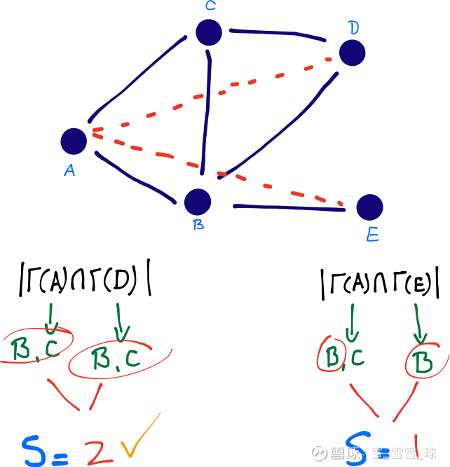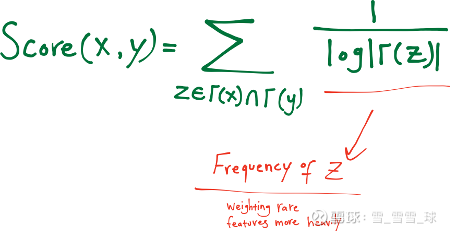3、优先连接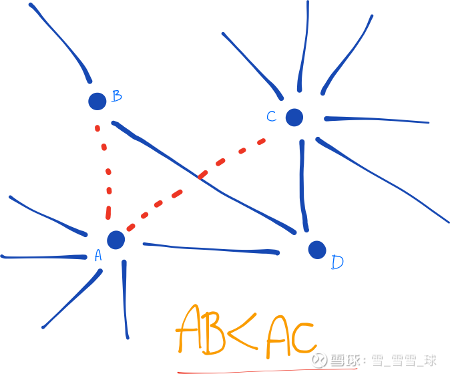（四）链路预测 - Neo4j 图算法库

（2）优先连接算法：计算每个节点的度数的乘积。

（3）资源分配算法：计算共同邻居的度数分之一，并求和。

（4）共同社区算法：利用社区发现算法，检查两个节点是否处于同一个社区。

（5）总邻居算法：计算两个节点所拥有的不同邻居的数目。

UNWIND [["A", "C"], ["A", "B"], ["B", "D"], ["B", "C"], ["B", "E"], ["C", "D"]] AS pairMERGE (n1:Node {name: pair})MERGE (n2:Node {name: pair})MERGE (n1)-[:FRIENDS]-(n2)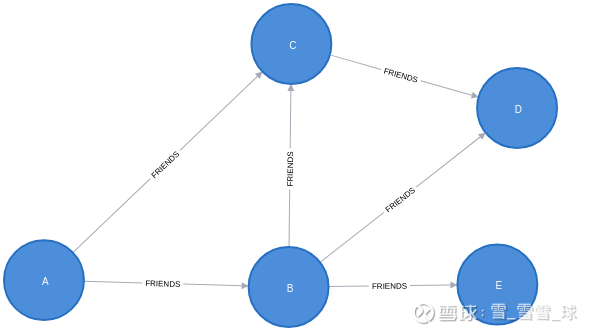neo4j> MATCH (a:Node {name: 'A'}) MATCH (d:Node {name: 'D'}) RETURN algo.linkprediction.commonNeighbors(a, d);+-------------------------------------------+| algo.linkprediction.commonNeighbors(a, d) |+-------------------------------------------+| 2.0 |+-------------------------------------------+1 row available after 97 ms, consumed after another 15 ms

neo4j> MATCH (a:Node {name: 'A'}) MATCH (e:Node {name: 'E'}) RETURN algo.linkprediction.commonNeighbors(a, e);+-------------------------------------------+| algo.linkprediction.commonNeighbors(a, e) |+-------------------------------------------+| 1.0 |+-------------------------------------------+

neo4j> WITH {direction: "BOTH", relationshipQuery: "FRIENDS"} AS config MATCH (a:Node {name: 'A'}) MATCH (e:Node {name: 'E'}) RETURN algo.linkprediction.commonNeighbors(a, e, config) AS score;+-------+| score |+-------+| 1.0 |+-------+

neo4j> MATCH (a:Node {name: 'A'}) MATCH (d:Node {name: 'D'}) RETURN algo.linkprediction.preferentialAttachment(a, d) AS score;+-------+| score |+-------+| 4.0 |+-------+

（五）链路预测所得的分数有何用？

1、直接使用指标

2、有监督学习

（1）具体要使用什么机器学习模型？

（2）如何将数据分成训练集和测试集？

（一）机器学习模型

（二）训练集和测试集

# negative examples = (# nodes)² - (# relationships) - (# nodes)

（三）代码教程：链路预测实战

1、录入引用数据库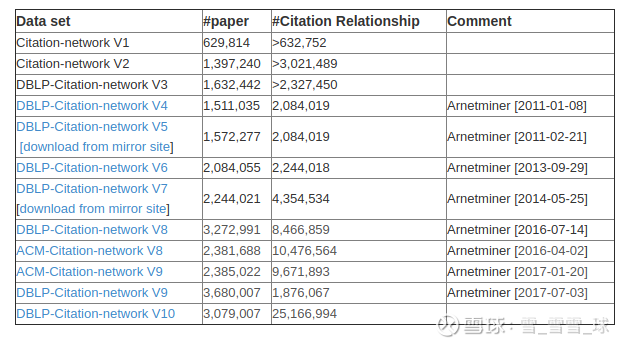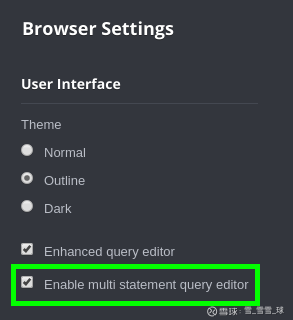// Create constraintsCREATE CONSTRAINT ON (a:Article) ASSERT a.index IS UNIQUE;CREATE CONSTRAINT ON (a:Author) ASSERT a.name IS UNIQUE;CREATE CONSTRAINT ON (v:Venue) ASSERT v.name IS UNIQUE;// Import data from JSON files using the APOC libraryCALL apoc.periodic.iterate( 'UNWIND ["dblp-ref-0.json", "dblp-ref-1.json", "dblp-ref-2.json", "dblp-ref-3.json"] AS file CALL apoc.load.json("网页链接 + file) YIELD value WITH value RETURN value', 'MERGE (a:Article {index:value.id}) SET a += apoc.map.clean(value,["id","authors","references", "venue"],) WITH a, value.authors as authors, value.references AS citations, value.venue AS venue MERGE (v:Venue {name: venue}) MERGE (a)-[:VENUE]->(v) FOREACH(author in authors | MERGE (b:Author{name:author}) MERGE (a)-[:AUTHOR]->(b)) FOREACH(citation in citations | MERGE (cited:Article {index:citation}) MERGE (a)-[:CITED]->(cited))', {batchSize: 1000, iterateList: true});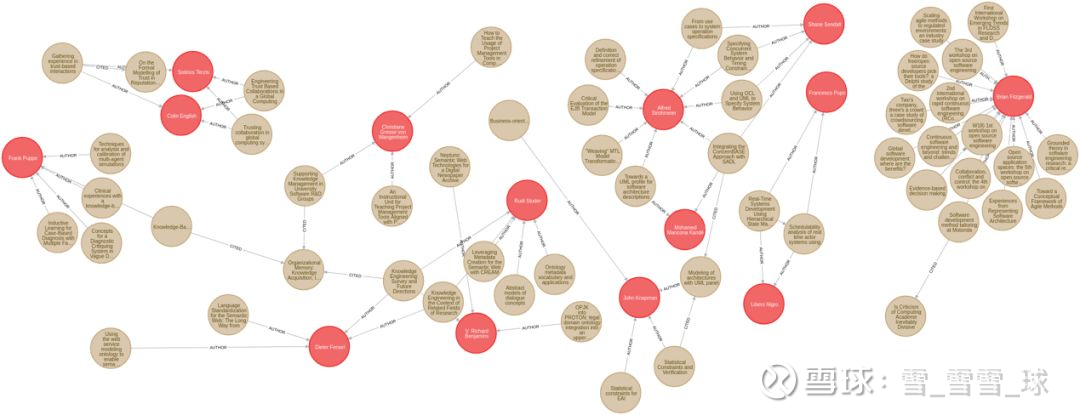2、搭建共同作者图

MATCH (a1)(a2:Author)WITH a1, a2, paperORDER BY a1, paper.yearWITH a1, a2, collect(paper).year AS year, count(*) AS collaborationsMERGE (a1)-[coauthor:CO_AUTHOR {year: year}]-(a2)SET coauthor.collaborations = collaborations;

（1）年份属性，指合作者们共同完成的第一篇文章的出版年份

（2）合作属性，指作者们合作过多少篇文章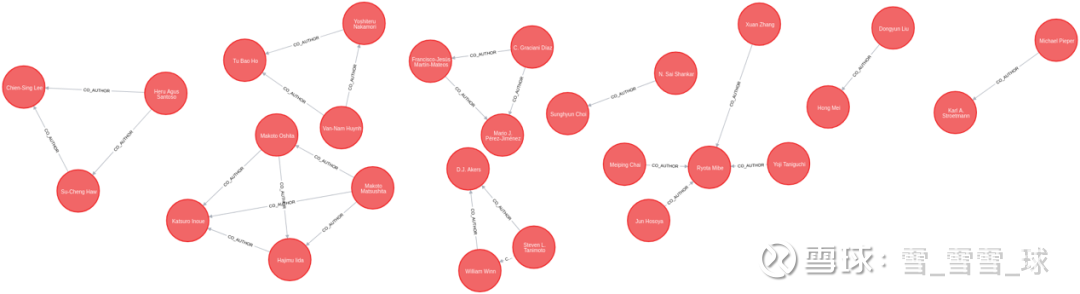Neo4j 中的共同作者

3、训练和测试数据集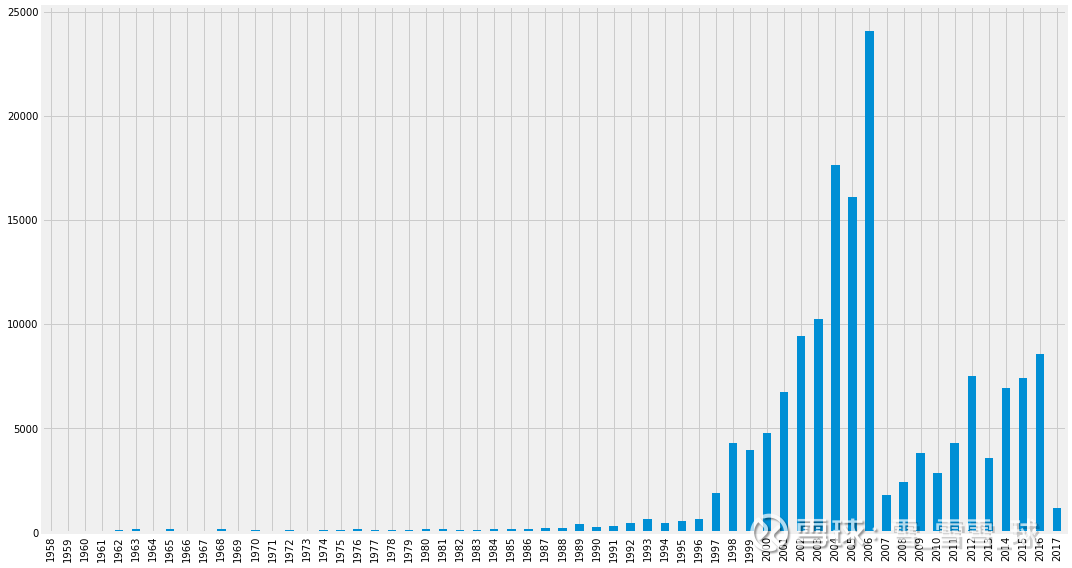（每年的合作数分布图）

MATCH (a)-[r:CO_AUTHOR]->(b) WHERE r.year

MATCH (a)-[r:CO_AUTHOR]->(b) WHERE r.year >= 2006MERGE (a)-[:CO_AUTHOR_LATE {year: r.year}]-(b);

# negative examples = (# nodes)² - (# relationships) - (# nodes)

MATCH (author:Author)WHERE (author)-[:CO_AUTHOR_EARLY]-()MATCH (author)-[:CO_AUTHOR_EARLY*2..3]-(other)WHERE not((author)-[:CO_AUTHOR_EARLY]-(other))RETURN id(author) AS node1, id(other) AS node2

4、Py2neo, pandas, scikit-learn

pip install py2neo==4.1.3 pandas sklearn

（1）py2neo驱动程序使数据科学家能够轻松地将Neo4j与Python数据科学生态系统中的工具相结合。我们将使用该库对Neo4j执行Cypher查询。

（2）pandas是BSD许可的开放源代码库，为Python编程语言提供了高性能、易于使用的数据结构和数据分析工具。

（3）scikit-learn是一个非常受欢迎的机器学习库。我们将使用该库来构建我们的机器学习模型。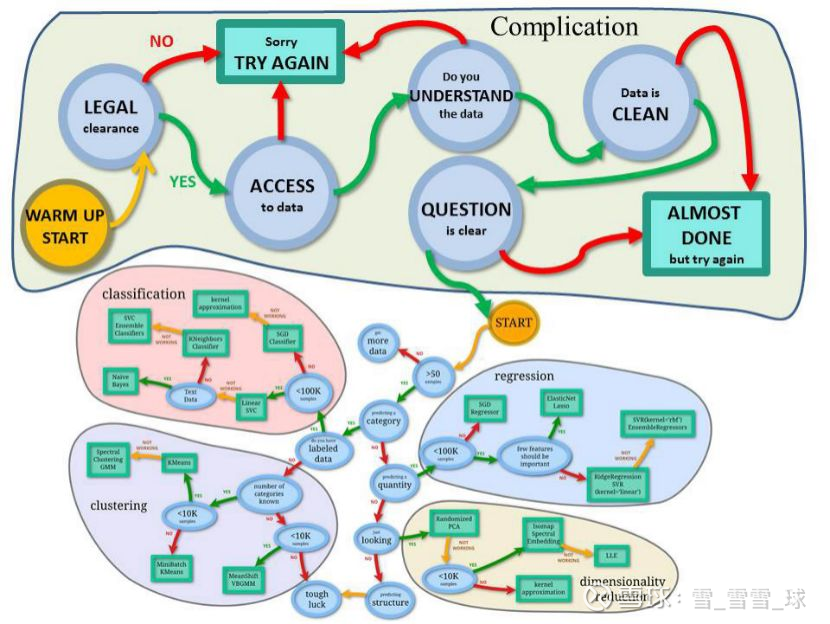（Scikit-Learn workflow 拓展版，来源网络）

from py2neo import Graphimport pandas as pdgraph = Graph("bolt://localhost", auth=("neo4j", "neo4jPassword"))

5、搭建我们的训练和测试集

# Find positive examplestrain_existing_links = graph.run("""MATCH (author:Author)-[:CO_AUTHOR_EARLY]->(other:Author)RETURN id(author) AS node1, id(other) AS node2, 1 AS label""").to_data_frame()# Find negative examplestrain_missing_links = graph.run("""MATCH (author:Author)WHERE (author)-[:CO_AUTHOR_EARLY]-()MATCH (author)-[:CO_AUTHOR_EARLY*2..3]-(other)WHERE not((author)-[:CO_AUTHOR_EARLY]-(other))RETURN id(author) AS node1, id(other) AS node2, 0 AS label""").to_data_frame()# Remove duplicatestrain_missing_links = train_missing_links.drop_duplicates()# Down sample negative examplestrain_missing_links = train_missing_links.sample( n=len(train_existing_links))# Create DataFrame from positive and negative examplestraining_df = train_missing_links.append( train_existing_links, ignore_index=True)training_df['label'] = training_df['label'].astype('category')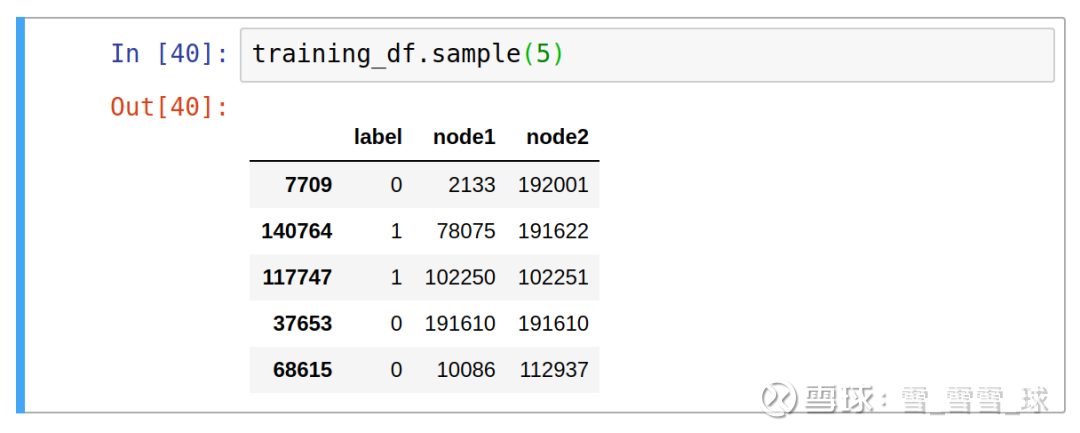一个测试数据集的例子

# Find positive examplestest_existing_links = graph.run("""MATCH (author:Author)-[:CO_AUTHOR_LATE]->(other:Author)RETURN id(author) AS node1, id(other) AS node2, 1 AS label""").to_data_frame()# Find negative examplestest_missing_links = graph.run("""MATCH (author:Author)WHERE (author)-[:CO_AUTHOR_LATE]-()MATCH (author)-[:CO_AUTHOR_LATE*2..3]-(other)WHERE not((author)-[:CO_AUTHOR_LATE]-(other))RETURN id(author) AS node1, id(other) AS node2, 0 AS label""").to_data_frame()# Remove duplicates test_missing_links = test_missing_links.drop_duplicates()# Down sample negative examplestest_missing_links = test_missing_links.sample(n=len(test_existing_links))# Create DataFrame from positive and negative examplestest_df = test_missing_links.append( test_existing_links, ignore_index=True)test_df['label'] = test_df['label'].astype('category')

6、选择机器学习算法

from sklearn.ensemble import RandomForestClassifierclassifier = RandomForestClassifier(n_estimators=30, max_depth=10, random_state=0)

7、生成链接预测特征

def apply_graphy_features(data, rel_type): query = """ UNWIND \$pairs AS pair MATCH (p1) WHERE id(p1) = pair.node1 MATCH (p2) WHERE id(p2) = pair.node2 RETURN pair.node1 AS node1, pair.node2 AS node2, algo.linkprediction.commonNeighbors( p1, p2, {relationshipQuery: \$relType}) AS cn, algo.linkprediction.preferentialAttachment( p1, p2, {relationshipQuery: \$relType}) AS pa, algo.linkprediction.totalNeighbors( p1, p2, {relationshipQuery: \$relType}) AS tn """ pairs = [{"node1": pair, "node2": pair} for pair in data[["node1", "node2"]].values.tolist()] params = {"pairs": pairs, "relType": rel_type} features = graph.run(query, params).to_data_frame() return pd.merge(data, features, on = ["node1", "node2"])

training_df = apply_graphy_features(training_df, "CO_AUTHOR_EARLY")test_df = apply_graphy_features(test_df, "CO_AUTHOR")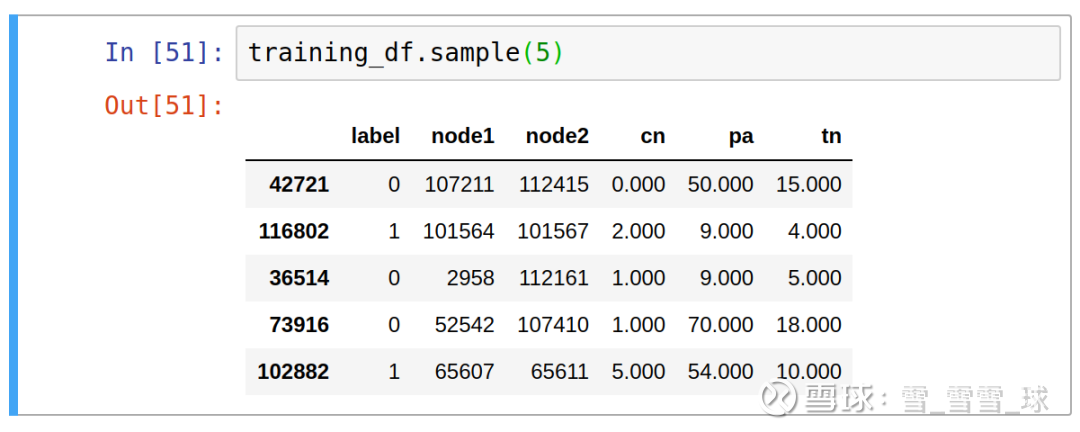columns = ["cn", "pa", "tn"]X = training_df[columns]y = training_df["label"]classifier.fit(X, y)

8、评估模型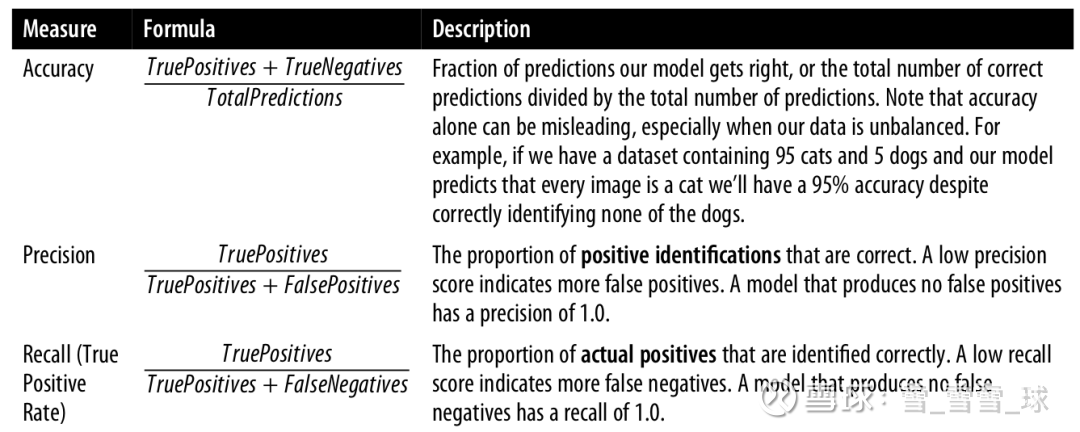from sklearn.metrics import recall_scorefrom sklearn.metrics import precision_scorefrom sklearn.metrics import accuracy_scoredef evaluate_model(predictions, actual): accuracy = accuracy_score(actual, predictions) precision = precision_score(actual, predictions) recall = recall_score(actual, predictions) metrics = ["accuracy", "precision", "recall"] values = [accuracy, precision, recall] return pd.DataFrame(data={'metric': metrics, 'value': values})def feature_importance(columns, classifier): features = list(zip(columns, classifier.feature_importances_)) sorted_features = sorted(features, key = lambda x: x*-1)
keys = [value for value in sorted_features] values = [value for value in sorted_features] return pd.DataFrame(data={'feature': keys, 'value': values})

predictions = classifier.predict(test_df[columns])y_test = test_df["label"]evaluate_model(predictions, y_test)（准确率，精准度，召回度）

feature_importance(columns, classifier)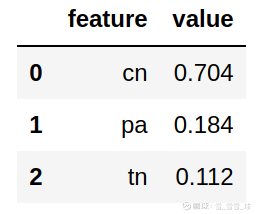（特征重要度）

9、三角形与聚类系数

CALL algo.triangleCount('Author', 'CO_AUTHOR_EARLY', { write:true, writeProperty:'trianglesTrain', clusteringCoefficientProperty:'coefficientTrain'});

CALL algo.triangleCount('Author', 'CO_AUTHOR', { write:true, writeProperty:'trianglesTest',   clusteringCoefficientProperty:'coefficientTest'});

def apply_triangles_features(data,triangles_prop,coefficient_prop): query = """ UNWIND \$pairs AS pair MATCH (p1) WHERE id(p1) = pair.node1 MATCH (p2) WHERE id(p2) = pair.node2 RETURN pair.node1 AS node1, pair.node2 AS node2, apoc.coll.min([p1[\$triangles], p2[\$triangles]]) AS minTriangles, apoc.coll.max([p1[\$triangles], p2[\$triangles]]) AS maxTriangles, apoc.coll.min([p1[\$coefficient], p2[\$coefficient]]) AS minCoeff, apoc.coll.max([p1[\$coefficient], p2[\$coefficient]]) AS maxCoeff """ pairs = [{"node1": pair, "node2": pair} for pair in data[["node1", "node2"]].values.tolist()] params = {"pairs": pairs, "triangles": triangles_prop, "coefficient": coefficient_prop} features = graph.run(query, params).to_data_frame() return pd.merge(data, features, on = ["node1", "node2"])

training_df = apply_triangles_features(training_df, "trianglesTrain", "coefficientTrain")test_df = apply_triangles_features(test_df, "trianglesTest", "coefficientTest")

columns = [ "cn", "pa", "tn", "minTriangles", "maxTriangles", "minCoeff", "maxCoeff"]X = training_df[columns]y = training_df["label"]classifier.fit(X, y)predictions = classifier.predict(test_df[columns])y_test = test_df["label"]display(evaluate_model(predictions, y_test))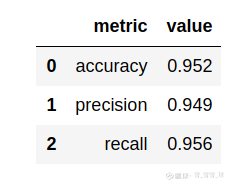（准确率，精准度，召回度）

display(feature_importance(columns, classifier))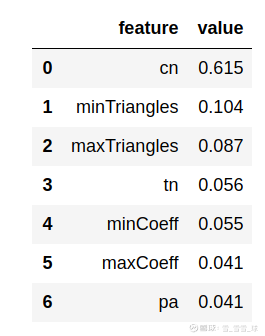（特征重要度）

（1）还有其他可添加的特征吗？这些特征能帮助我们创建更高准确性的模型吗？也许其他社区检测甚至中心算法也可能会有所帮助？

（2）目前，图形算法库中的链接预测算法仅适用于单零件图（两个节点的标签相同的图），该算法基于节点的拓扑；如果我们尝试将其应用于具有不同标签的节点（这些节点可能具有不同的拓扑），这就意味着此算法无法很好地发挥作用，所以目前也在考虑添加适用于其他图表的链接预测算法的版本，也欢迎大家在Github上一起交流。

Github地址：

（*本文为AI科技大本营编译文章，转载请微信联系 1092722531）2019-12-03 19:33 雪球 转发：0 回复：0 喜欢：5